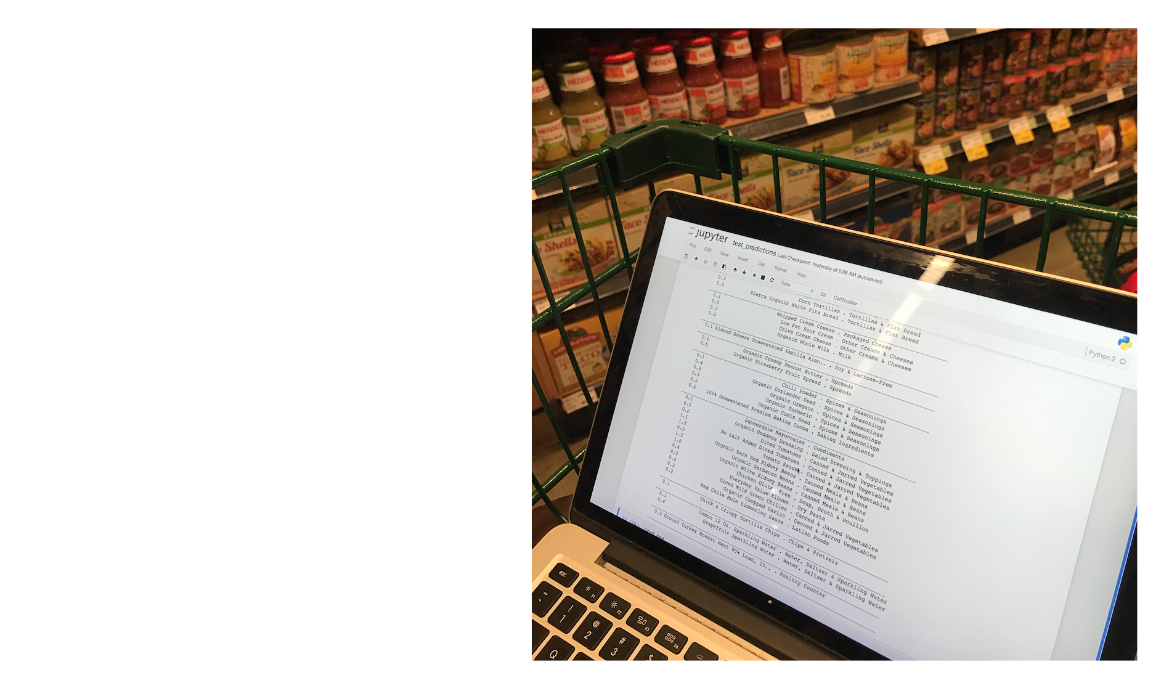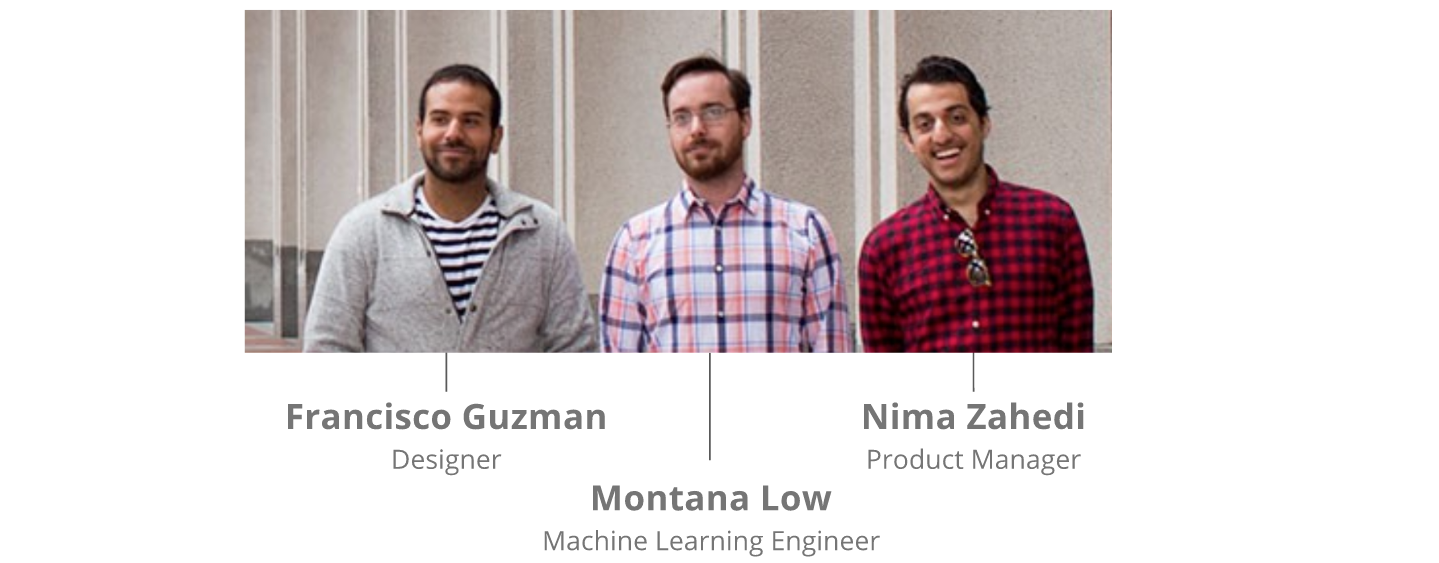使用 Emoji（而非数学知识）进行深度学习

Instacart 的目标是帮助客户从数百个零售商合作伙伴处订购上百万种产品。我们“购物顾问”团队中成千上万的成员必须能准确地在数千个店铺快速找到客户想要购买的商品。因此必须想方设法让这一过程尽可能快速。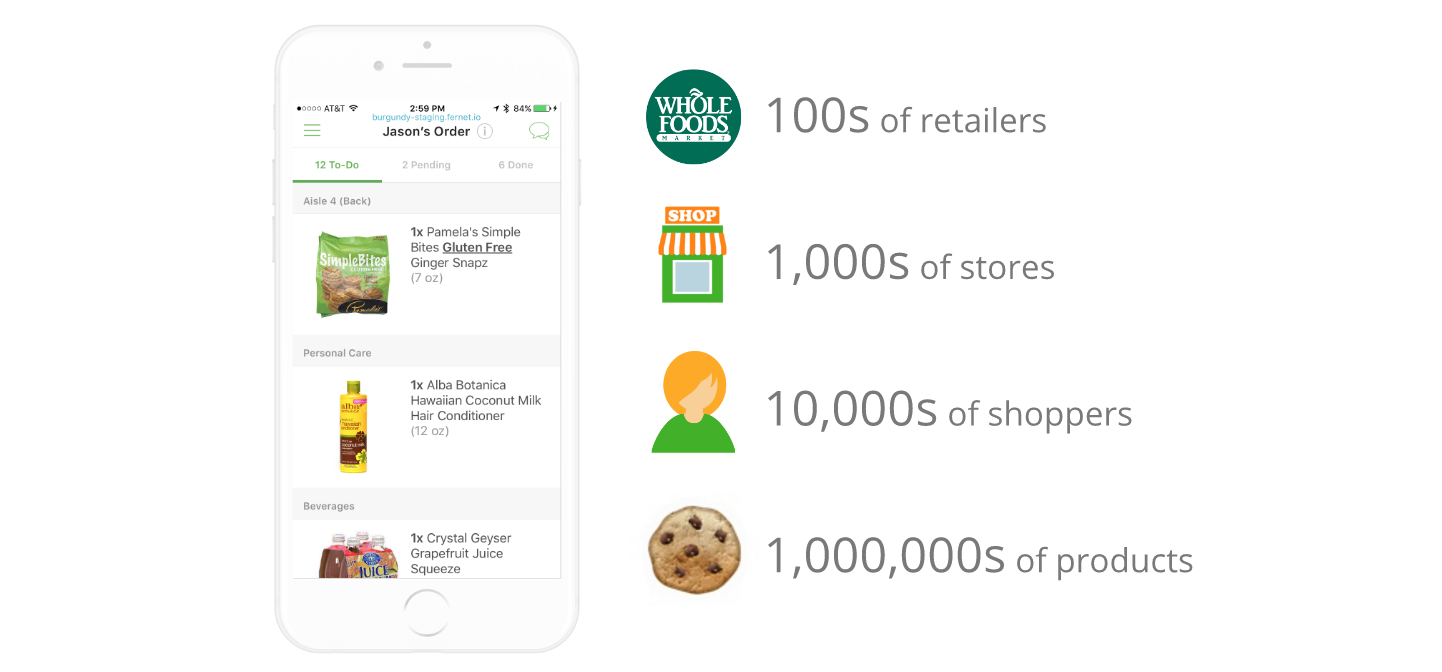深度学习技术“加持”的购物体验

深度学习入门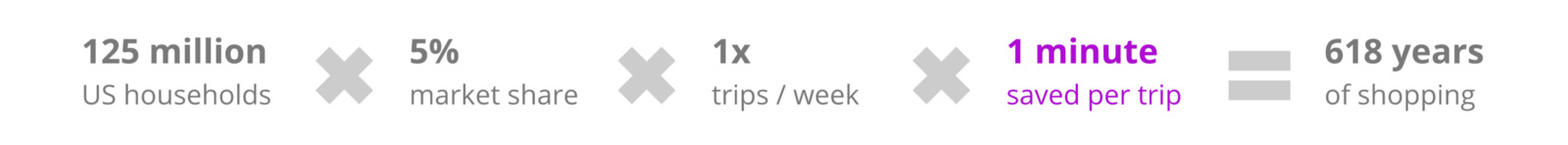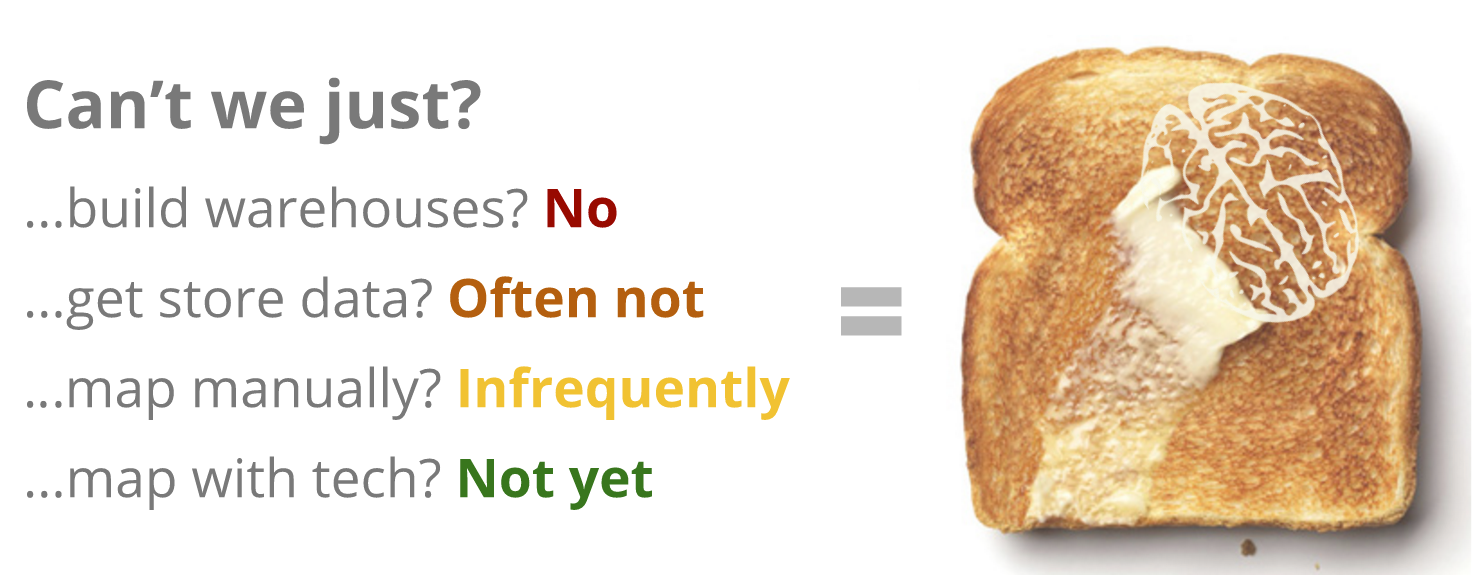进行过的测试

• Control（红色）：按照部门随机排序，同部门内商品按字母顺序排序；
• Human（绿色）：使用店铺陈列布局按照店铺走道排序，同走道内商品按字母顺序排序；
• TSP（青色）：使用跨部门商品挑选平均时间构建的“巡回推销员”解决方案，同部门内商品按字母顺序排序；
• Deep（紫色）：最终的深度学习架构，直接对每批中的不同商品进行排序。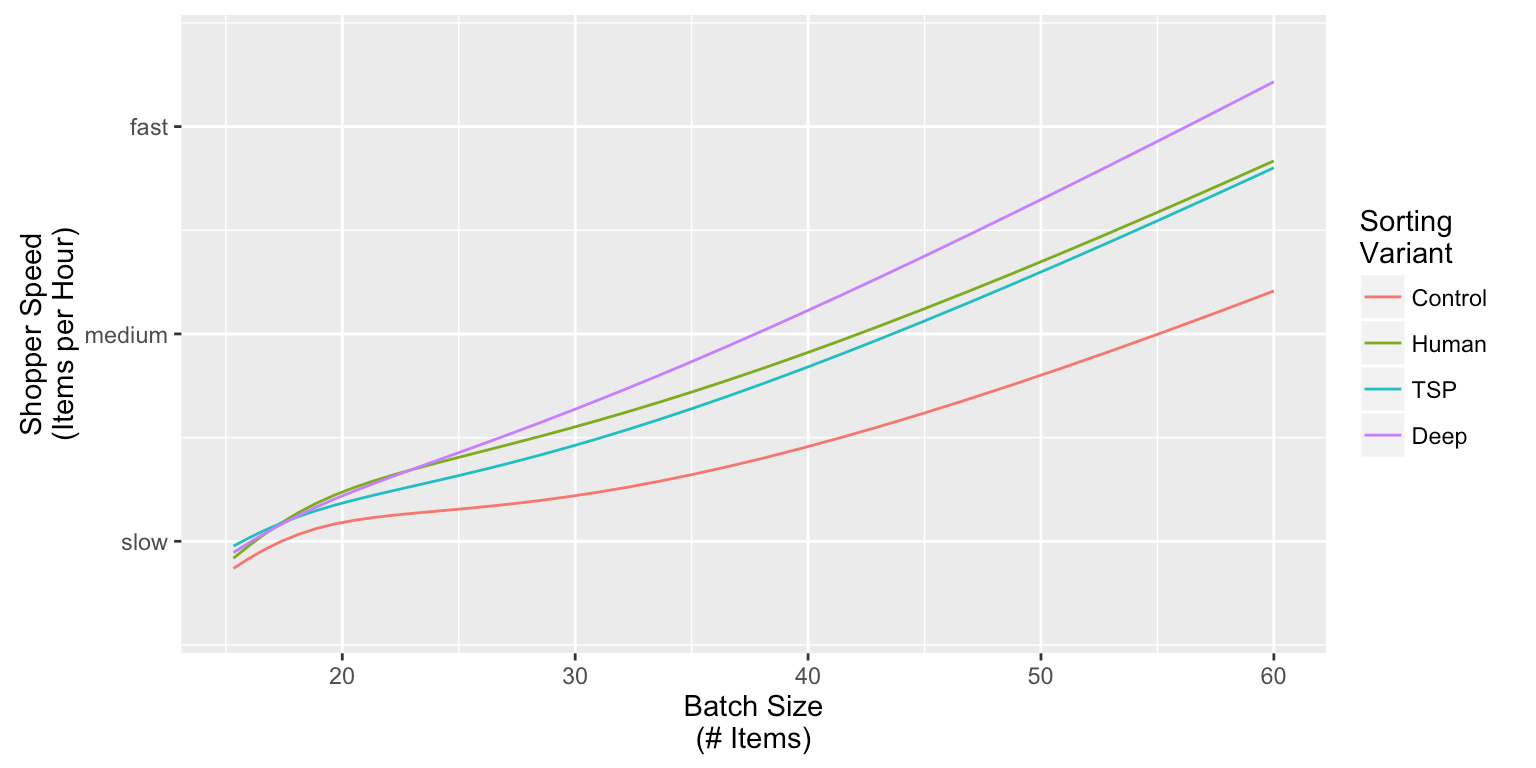Control 排序方式表现最差（意料之中）。TSP 和 Human 的表现好很多，但从统计结果来看两者没有太大差异。

通过 Keras 进行清单排序

问题定义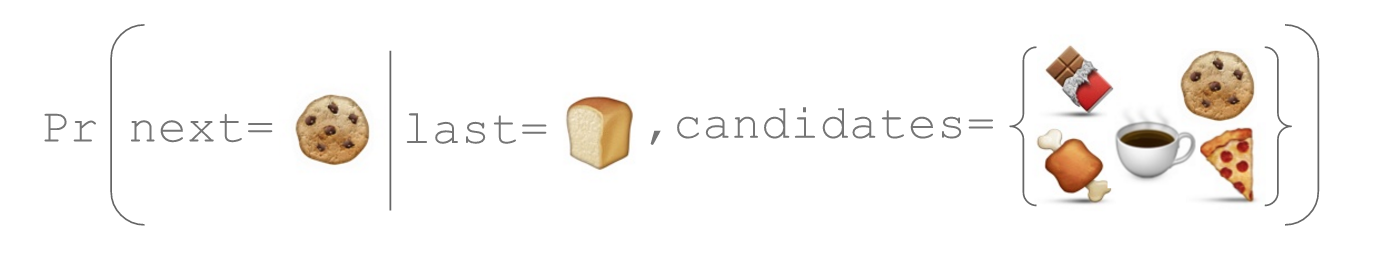初始架构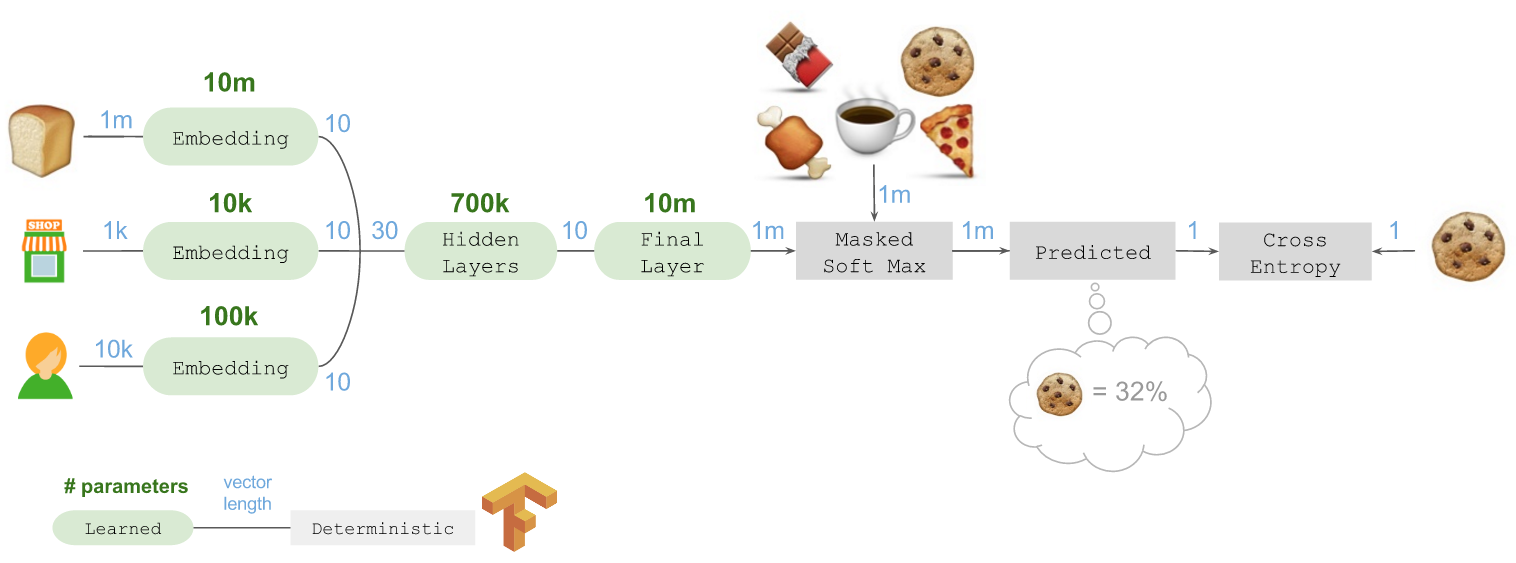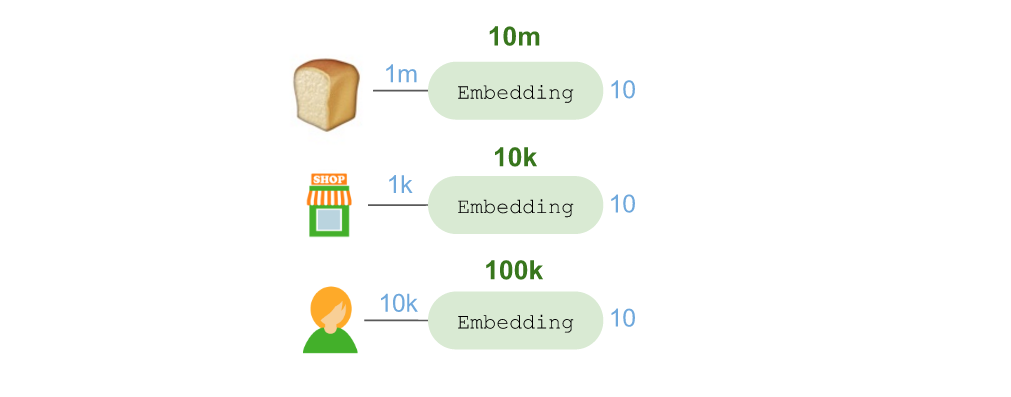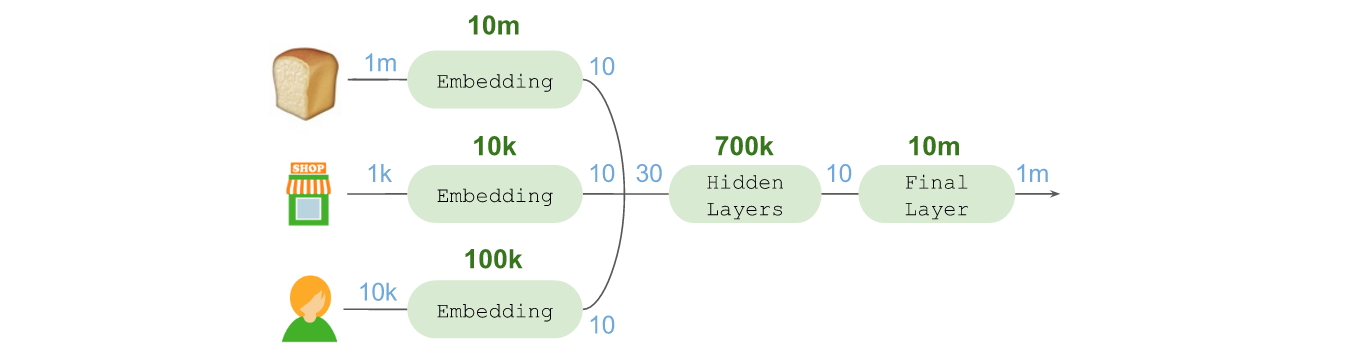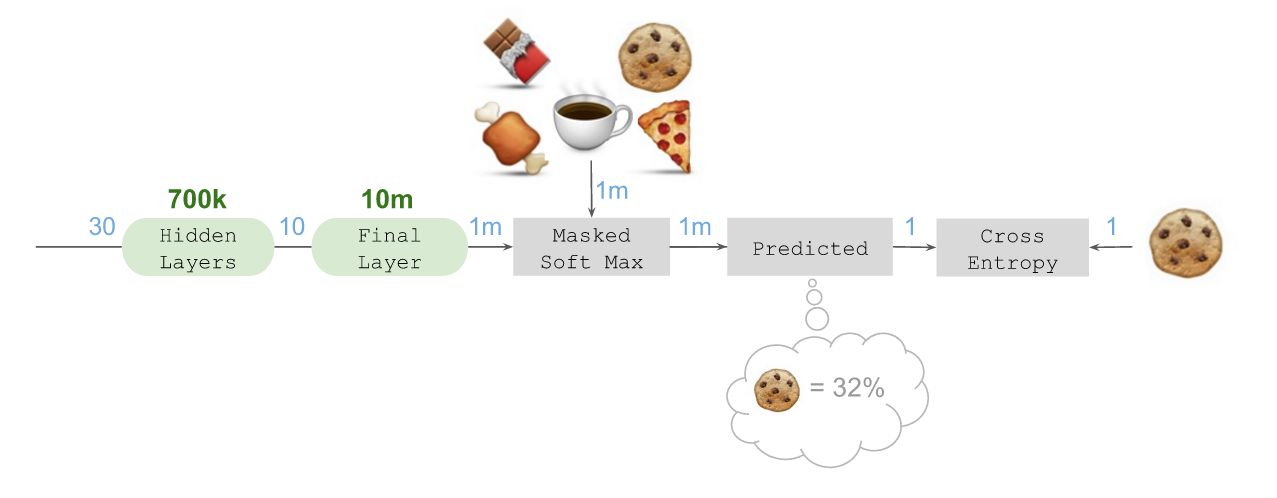Keras 代码

from keras.models import Model
from keras.layers.core import Dense, Reshape, Lambda
from keras.layers import Input, Embedding, merge
from keras import backend as K

# Number of product IDs available
N_products = 1000000
N_stores = 1000
N_shoppers = 10000

# Integer IDs representing 1-hot encodings
prior_in = Input(shape=(1,))
store_in = Input(shape=(1,))
shopper_in = Input(shape=(1,))

# Dense N-hot encoding for candidate products
candidates_in = Input(shape=(N_products,))

# Embeddings
prior = Embedding(N_products, 10)(prior_in)
store = Embedding(N_stores, 10)(store_in)
shopper = Embedding(N_shoppers, 10)(shopper_in)

# Reshape and merge all embeddings together
reshape = Reshape(target_shape=(10,))
combined = merge([reshape(prior), reshape(store), reshape(shopper)],
mode='concat')

# Hidden layers
hidden_1 = Dense(1024, activation='relu')(combined)
hidden_2 = Dense(512, activation='relu')(hidden_1)
hidden_3 = Dense(256, activation='relu')(hidden_2)
hidden_4 = Dense(10, activation='linear')(hidden_3)

# Final 'fan-out' into the space of future products
final = Dense(N_products, activation='linear')(hidden_4)

# Ensure we do not overflow when we exponentiate
final = Lambda(lambda x: x - K.max(x))(final)

# Masked soft-max using Lambda and merge-multiplication
exponentiate = Lambda(lambda x: K.exp(x))(final)
masked = merge([exponentiate, candidates_in], mode='mul')
predicted = Lambda(lambda x: x / K.sum(x))(masked)

# Compile with categorical crossentropy and adam
mdl = Model(input=[prior_in, store_in, shopper_in, candidates_in],
output=predicted)
mdl.compile(loss='categorical_crossentropy',
metrics=['accuracy'])

initial_architecture.py 代码托管于? GitHub

检测和局限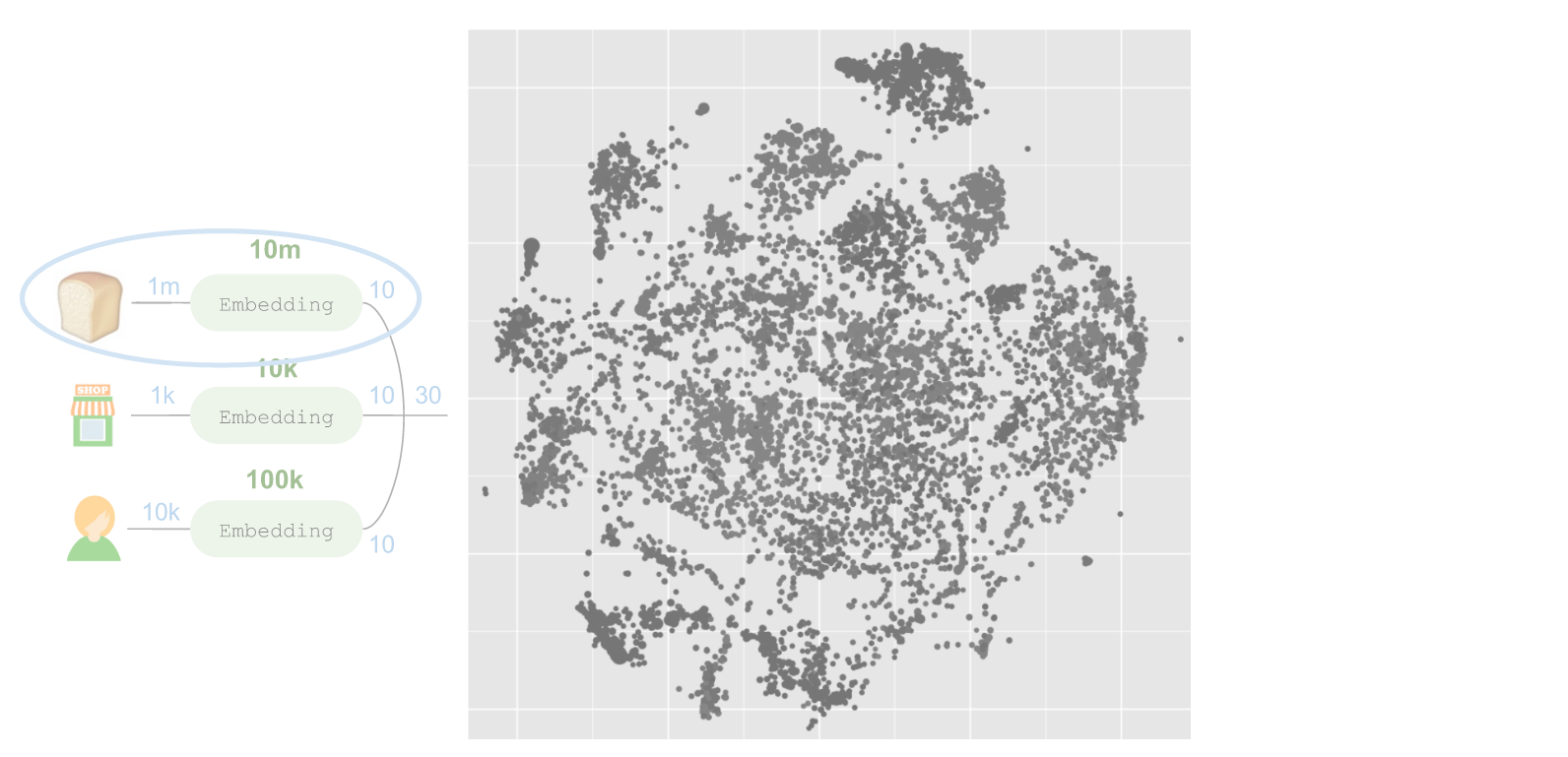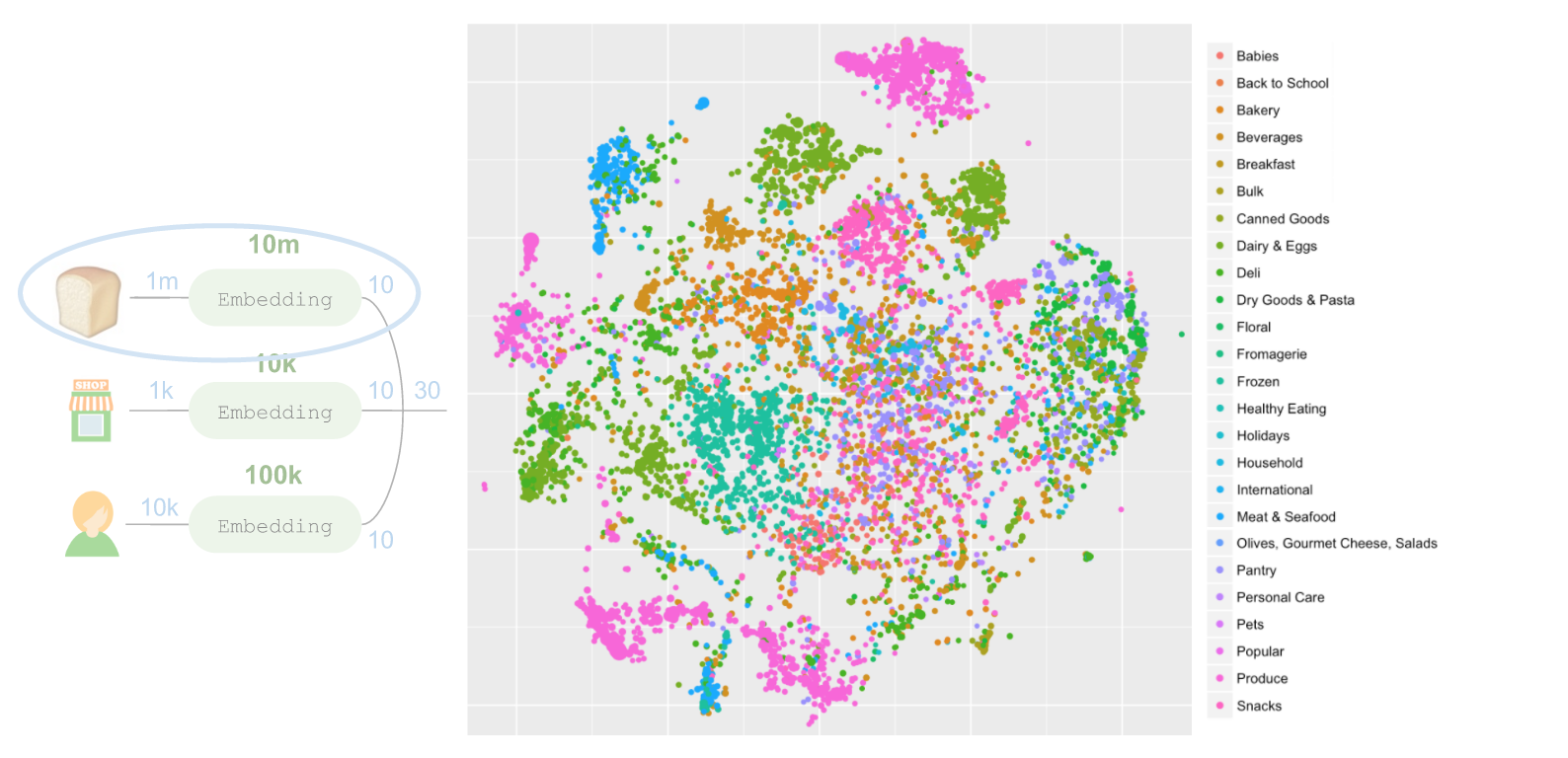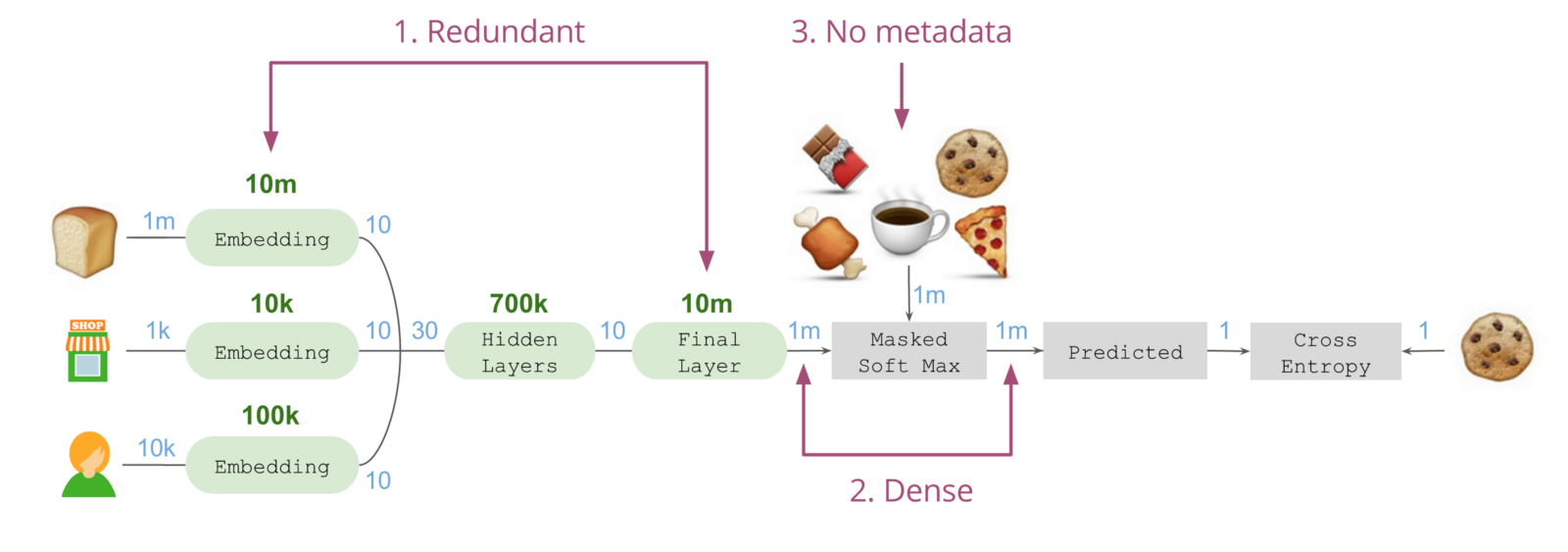最终架构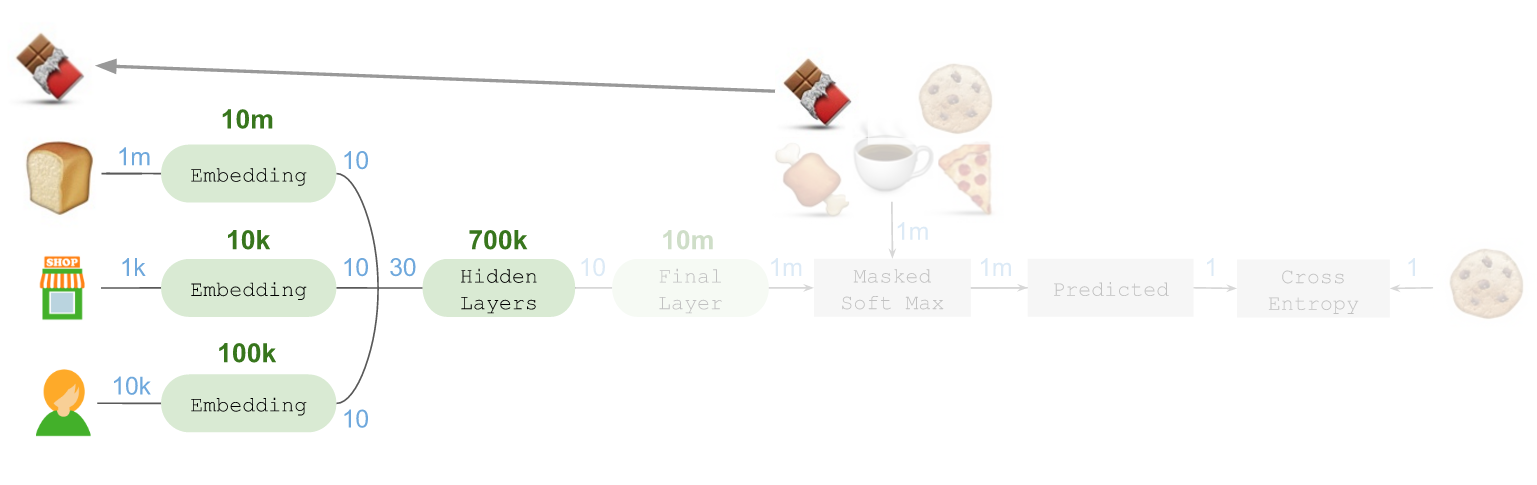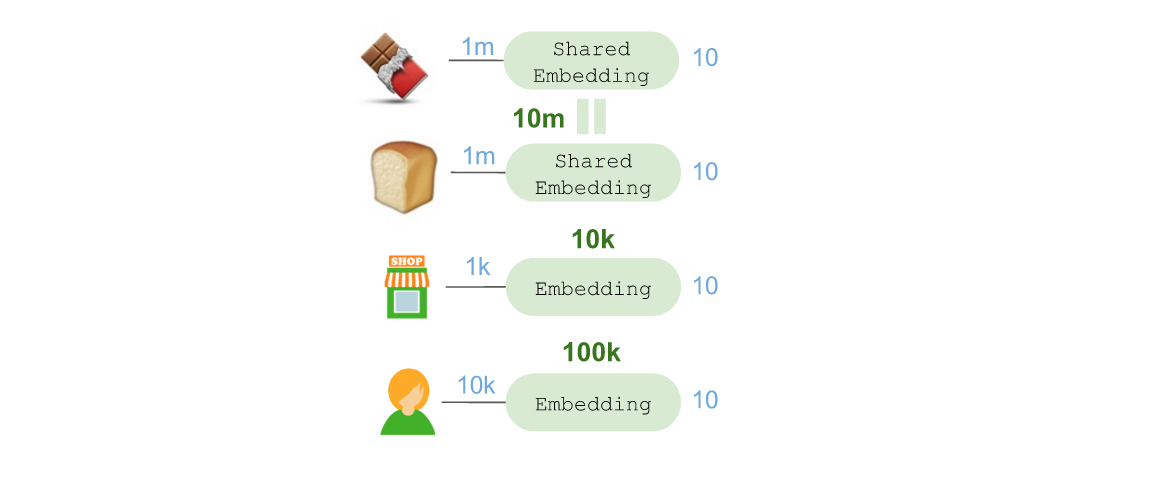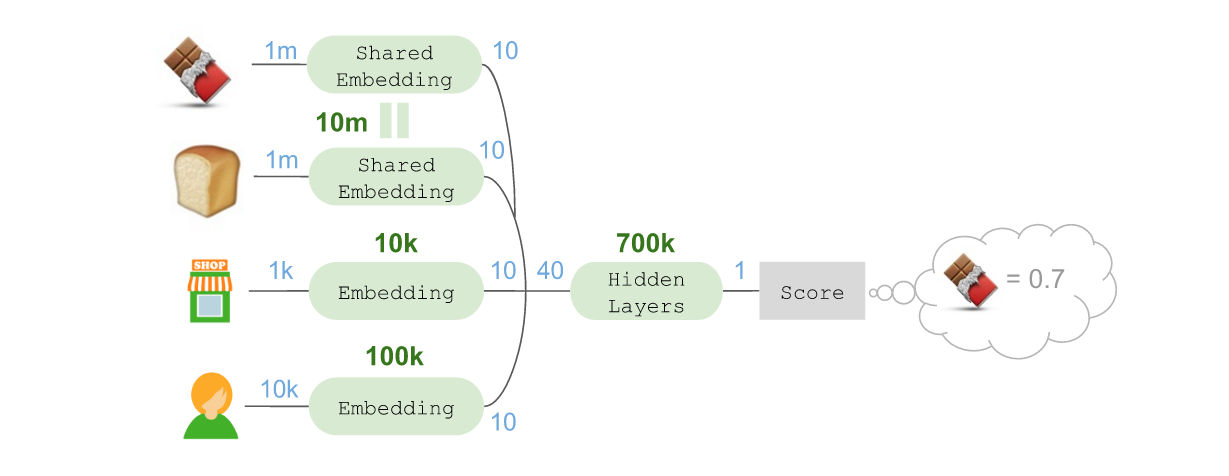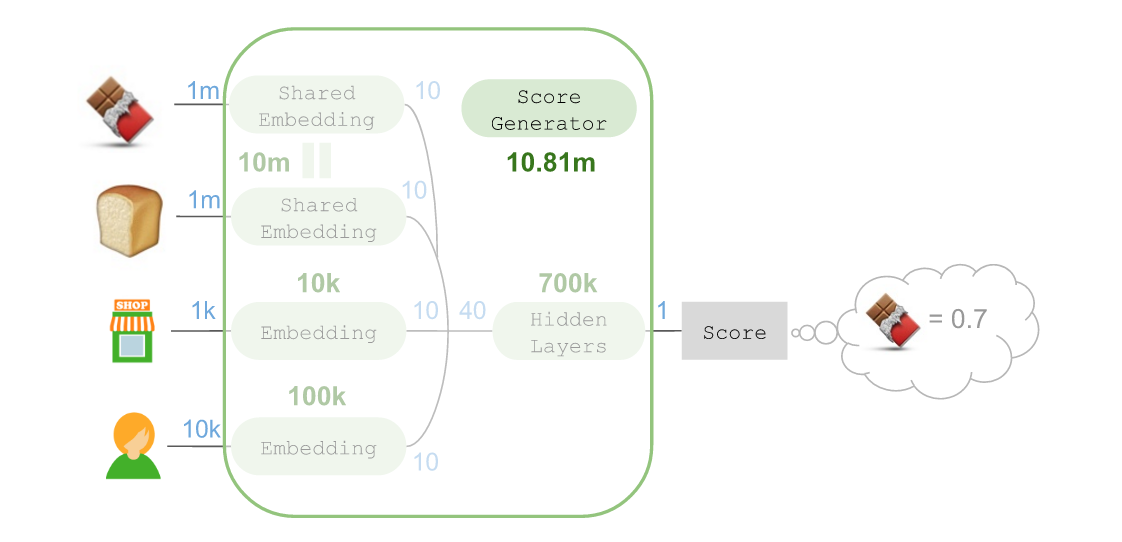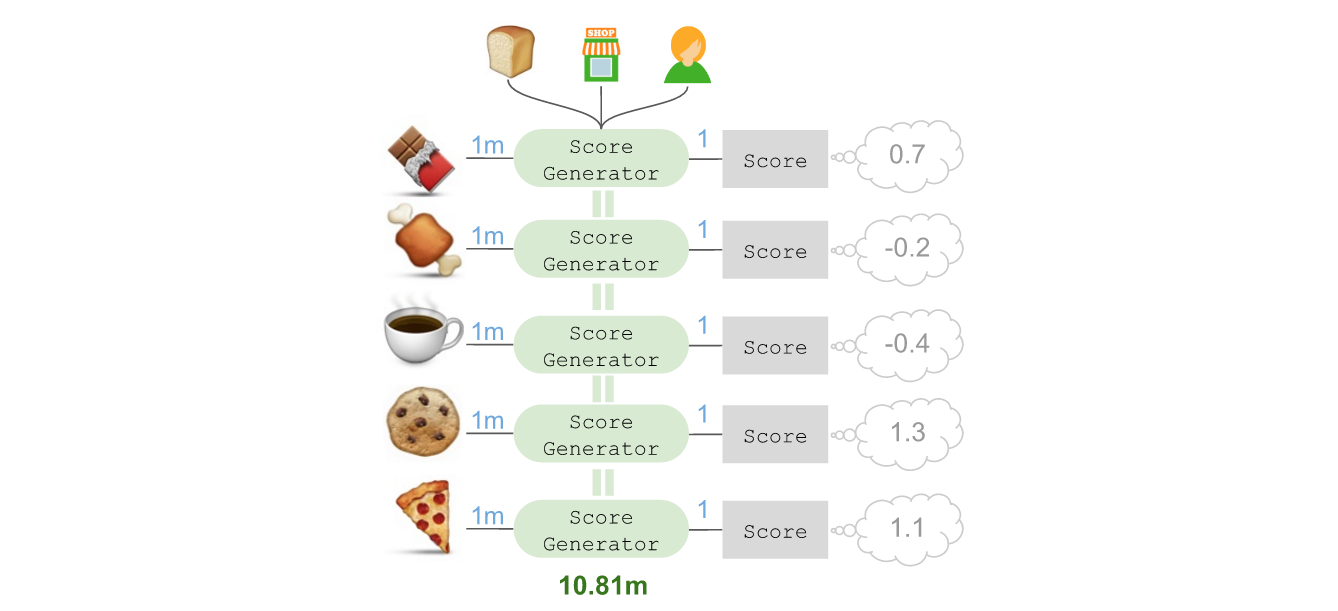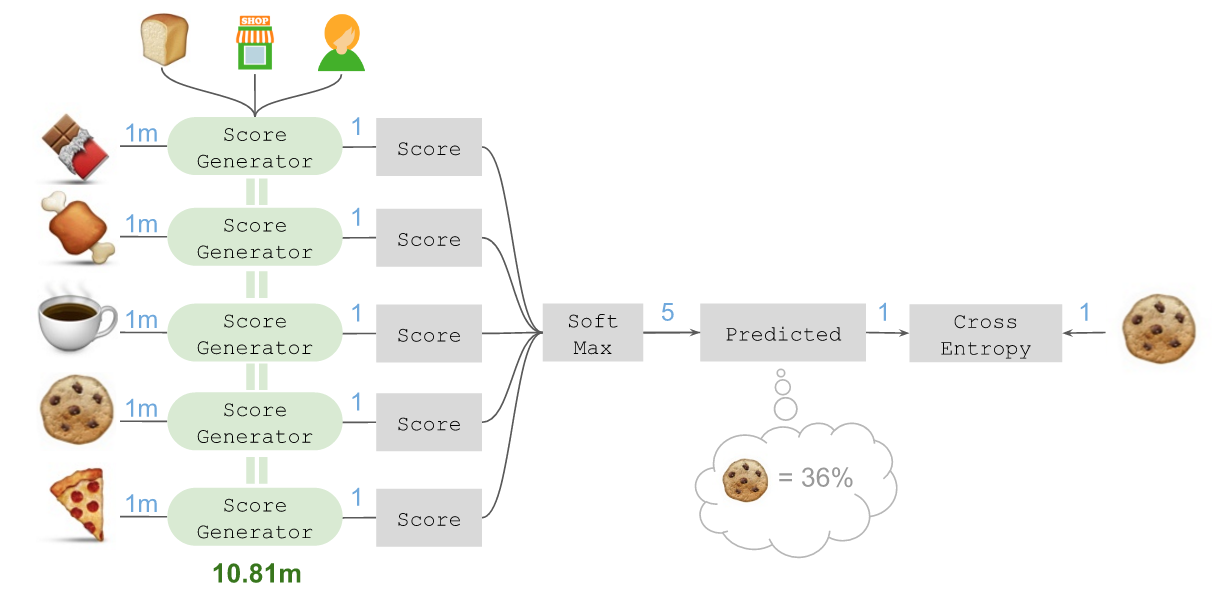最终结果